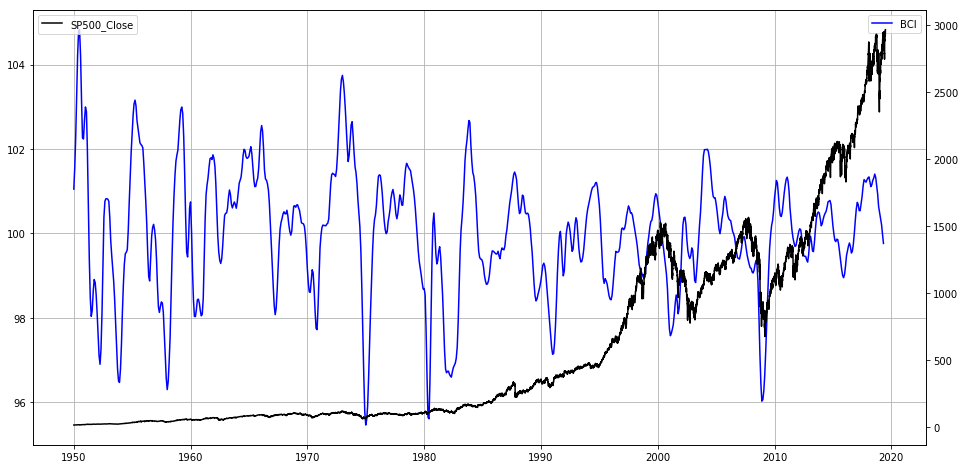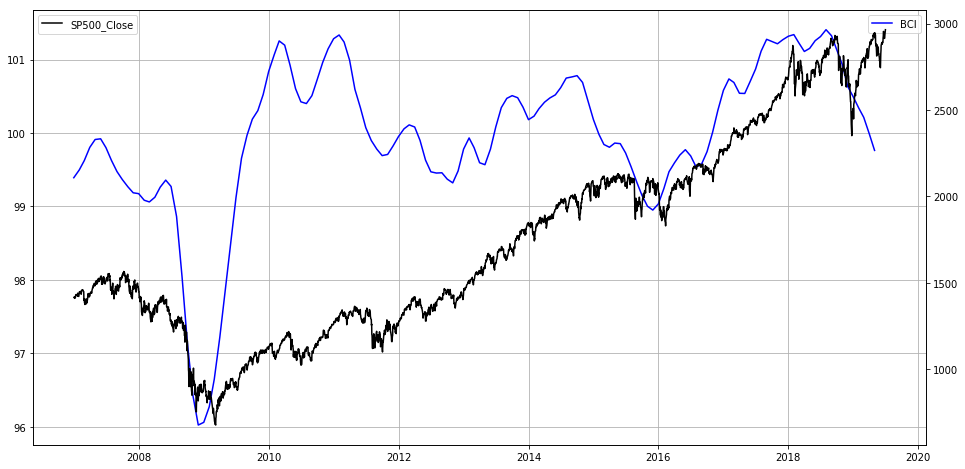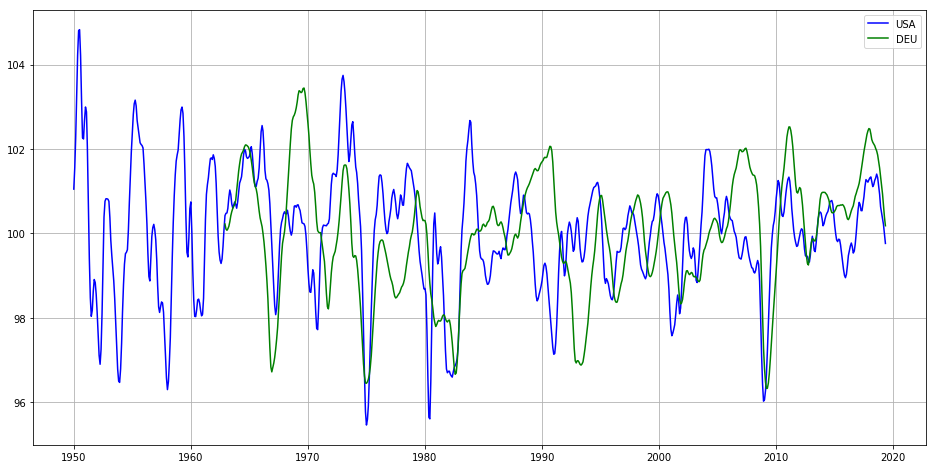My New Udemy Class: Fundamental Market Analysis with PythonFundamental Market Analysis with Python - Find Your Own Answers On What Is Going on in the Financial Markets

Hot off the Press!My New Book: "The Little Book of Fundamental Analysis: Hands-On Market Analysis with Python" is Out!

Grow Your Web Brand, Visibility & Traffic Organically5 Years of amunategui.github.Io and the Lessons I Learned from Growing My Online Community from the Ground Up

Introduction

Lets Explore the Business Confidence Index Together (BCI). This is a powerful leading indicator. Im starting from scratch so you can follow along.

Code

In :
from IPython.display import Image
Image(filename='viralml-book.png')
Out:Fundamental and Technical Indicators - Hands-On Market Analysis¶

Get the book: https://amzn.to/2DERG3d

More on: https://www.viralml.com/

Business Confidence Index (BCI)¶

This business confidence indicator provides information on future developments, based upon opinion surveys on developments in production, orders and stocks of finished goods in the industry sector. It can be used to monitor output growth and to anticipate turning points in economic activity. Numbers above 100 suggest an increased confidence in near future business performance, and numbers below 100 indicate pessimism towards future performance.

In :
%matplotlib inline
import matplotlib
import matplotlib.pyplot as plt
import io, base64, os, json, re
import pandas as pd
import numpy as np
import datetime
import warnings
warnings.filterwarnings('ignore')
In :
path_to_market_data = '/Users/manuel/Documents/financial-research/market-report/2019-07-02/'

In :
bci_df = pd.read_csv(path_to_market_data + 'DP_LIVE_02072019085313431.csv')
bci_df = bci_df[bci_df['LOCATION'] == 'USA']
bci_df['Date'] = bci_df['TIME'] + '-01'
bci_df['Date'] = pd.to_datetime(bci_df['Date'])
bci_df = bci_df.sort_values('Date', ascending=True) # sort in ascending date order
bci_df = bci_df[['Date','Value']]
bci_df.columns = ['Date', 'BCI']
print(np.min(bci_df['Date'] ),np.max(bci_df['Date'] ))
1950-01-01 00:00:00 2019-05-01 00:00:00
Out:
Date BCI
7673 1950-01-01 101.0515
7674 1950-02-01 101.5680
7675 1950-03-01 102.2565
7676 1950-04-01 103.2375
7677 1950-05-01 104.2263
In :
sp500_df = pd.read_csv(path_to_market_data + '^GSPC.csv')
sp500_df['Date'] = pd.to_datetime(sp500_df['Date'])
sp500_df = sp500_df[['Date','Adj Close']]
sp500_df.columns = ['Date', 'SP500_Close']
print(np.min(sp500_df['Date'] ),np.max(sp500_df['Date'] ))
sp500_df = sp500_df.sort_values('Date', ascending=True) # sort in ascending date order
1950-01-03 00:00:00 2019-07-01 00:00:00
Out:
Date SP500_Close
0 1950-01-03 16.66
1 1950-01-04 16.85
2 1950-01-05 16.93
3 1950-01-06 16.98
4 1950-01-09 17.08

Plot¶

In :
fig, ax = plt.subplots(figsize=(16, 8))

plt.plot(bci_df['Date'], bci_df['BCI'], label='BCI', color='blue')
plt.legend(loc='upper right')
plt.grid()

# Get second axis
ax2 = ax.twinx()

plt.plot(sp500_df['Date'],  sp500_df['SP500_Close'], label='SP500_Close',color='black')
plt.legend(loc='upper left')
plt.show()Let's zoom in¶

In :
bci_df_tmp = bci_df.copy()
sp500_df_tmp = sp500_df.copy()

cut_off_date = '2007-01-01'
bci_df_tmp = bci_df_tmp[bci_df_tmp['Date'] >= cut_off_date]
sp500_df_tmp = sp500_df_tmp[sp500_df_tmp['Date'] >= cut_off_date]

fig, ax = plt.subplots(figsize=(16, 8))
plt.plot(bci_df_tmp['Date'], bci_df_tmp['BCI'], label='BCI', color='blue')
plt.legend(loc='upper right')
plt.grid()

# Get second axis
ax2 = ax.twinx()

plt.plot(sp500_df_tmp['Date'],  sp500_df_tmp['SP500_Close'], label='SP500_Close',color='black')
plt.legend(loc='upper left')
plt.show()Let's Compare Different Countries¶

In :
bci_df = pd.read_csv(path_to_market_data + 'DP_LIVE_02072019085313431.csv')
set(bci_df['LOCATION'])
Out:
{'AUS',
'AUT',
'BEL',
'BRA',
'CAN',
'CHE',
'CHL',
'CHN',
'CZE',
'DEU',
'DNK',
'EA19',
'ESP',
'EST',
'FIN',
'FRA',
'G-7',
'GBR',
'GRC',
'HUN',
'IDN',
'IND',
'IRL',
'ISR',
'ITA',
'JPN',
'KOR',
'LTU',
'LUX',
'LVA',
'MEX',
'NLD',
'NOR',
'NZL',
'OECD',
'OECDE',
'POL',
'PRT',
'RUS',
'SVK',
'SVN',
'SWE',
'TUR',
'USA',
'ZAF'}
In :
bci_df = bci_df[bci_df['LOCATION'].isin(['USA','DEU'])]
bci_df['Date'] = bci_df['TIME'] + '-01'
bci_df['Date'] = pd.to_datetime(bci_df['Date'])
bci_df = bci_df.sort_values('Date', ascending=True)
bci_df = bci_df[['Date', 'Value','LOCATION']]
In :
fig, ax = plt.subplots(figsize=(16, 8))

bci_df[bci_df['LOCATION']=='USA']['Date']

plt.plot(bci_df[bci_df['LOCATION']=='USA']['Date'],
bci_df[bci_df['LOCATION']=='USA']['Value'], label='USA', color='blue')
plt.plot(bci_df[bci_df['LOCATION']=='DEU']['Date'],
bci_df[bci_df['LOCATION']=='DEU']['Value'], label='DEU', color='green')
plt.grid()
plt.legend(loc='upper right')
Out:
<matplotlib.legend.Legend at 0x11dc69ef0>Show Notes

(pardon typos and formatting -
these are the notes I use to make the videos)